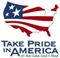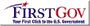Scientific Investigations Report 2006-5122

U.S. GEOLOGICAL SURVEY
Scientific Investigations Report 2006-5122

## Conversion Factors and Datums

### Conversion Factors

Multiply By To obtain
acre 4,047 square meter (m2)
acre-foot (acre-ft) 1,233 cubic meter (m3)
cubic foot (ft3) 0.02832 cubic meter (m3)
cubic foot per second (ft3/s) 0.02832 cubic meter per second (m3/s)
cubic foot per day (ft3/d) 0.02832 cubic meter per day (m3/d)
cubic foot per second per square mile [(ft3/s)/mi2] 0.01094 cubic meter per second per square kilometer [(m3/s)/km2]
curie (Ci) 37,000 megabecquerel (MBq)
foot (ft) 0.3048 meter (m)
foot per mile (ft/mi) 0.1894 meter per kilometer (m/km)
foot per day (ft/d) .3048 meter per day (m/d)
foot per year (ft/yr) .3048 meter per year (m/yr)
foot squared per day (ft2/d) 0.09290 meter squared per day (m2/d)
gallon (gal) 3.785 liter (L)
gallon per minute (gal/min) 0.06309 liter per second (L/s)
million gallons (Mgal) 3,785 cubic meter (m3)
inch per year (in/yr) 25.4 millimeter per year (mm/yr)
mile (mi) 1.609 kilometer (km)
picocurie per liter (pCi/L) 0.037 becquerel per liter (Bq/L)
square foot (ft2) 929.0 square centimeter (cm2)
square foot (ft2) 0.09290 square meter (m2)
square mile (mi2) 2.590 square kilometer (km2)

Temperature in degrees Celsius (°C) may be converted to degrees Fahrenheit (°F) as follows:

°F=(1.8×°C)+32

Transmissivity: The standard unit for transmissivity is cubic foot per day per square foot times feet of aquifer thickness [(ft3/d)/ft2]ft. In this report, the mathematically reduced form, foot squared per day (ft2/d), is used for convenience.

Specific conductance is given in microsiemens per centimeter at 25 degrees Celsius (µS/cm at 25°C).

Concentrations of chemical constituents in water are given either in milligrams per liter (mg/L) or micrograms per liter (µg/L).

### Datums

Vertical coordinate information is referenced to the National Geodetic Vertical Datum of 1929 (NGVD 29).

Horizontal coordinate information is referenced to North American Datum of 1927 (NAD 27).

Altitude, as used in this report, refers to distance above the vertical datum.U.S. Department of the Interior | U.S. Geological Survey